Refer to our Texas Go Math Grade 6 Answer Key Pdf to score good marks in the exams. Test yourself by practicing the problems from Texas Go Math Grade 6 Unit 5 Study Guide Review Answer Key.

Angles, Triangles, and Equations

Essential Question
How can you use angles, triangles, and equations to solve real-world problems?

Exercises

Tell whether a triangle can have sides with the given lengths. If it cannot, give an inequality that shows why not. (Lesson 15.1)

Question 1.
5 in., 12 in., 13 in.. ______
Find the sum of the two sides of the triangLe then compare to the third side.

5 + 12 > 13 add the two sides and compare to the third side
17 > 13 the sum of the two sides is greater than the third side

5 + 13 > 12 add the two sides and compare to the third side
18 > 12 the sum of the two sides is greater than the third side

13 + 12 > 5 add the two sides and compare to the third side
25 > 5 the sum of the two sides is greater than the third side

The given sides can be the side lengths of a triangle because the sum of two sides is greater than the third side.

Question 2.
4.5 ft, 5.5 ft, 11 ft. ______
Find the sum of the two sides of the triangle then compare to the third side.
4.5 + 5.5 > 11 add the two sides and compare to the third side
10 ≯ 11 the sum of the two sides is NOT greater than the third side

4.5 + 11 > 5.5 add the two sides and compare to the third side
15.5 > 5.5 the sum of the two sides is greater than the third side

11 + 5.5 > 4.5 add the two sides and compare to the third side
16.5 > 4.5 the sum of the two sides is greater than the third side

The given side lengths cannot be used for a triangle because there are two sides with a sum lesser than the third side.

Find each missing angle measure. Classify each triangle as acute, obtuse, or right. (Lesson 15.2)

Question 3.Determine the unknown angle.
38° + 82° + x = 180° add all the angle measurements
120° + x = 180° sum of the given inner angle measurements
120° + x – 120° = 180° – 120° subtract 120° from both sides of the equation
x = 60° measure of the unknown angle

The value of x is 60°.

Question 4.Determine the unknown angle.
41°+ x + 49° = 180° add all the angle measurements
90° + x = 180° sum of the given inner angle measurements
90°+ x – 90°= 180° – 90° subtract 90° from both sides of the equation
x = 90° measure of the unknown angle
The value of x is 90°.

Match each side length with its correct measure. Classify each triangle as scalene, isosceles, or equilateral. (Lesson 15.3)

Question 5.
The side lengths of triangle ABC are 6.4 ft, 10 ft, and 6.4 ft.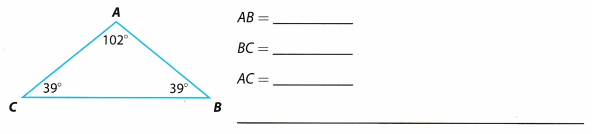Determine the side length of the triangle.
AB = 6.4 ft opposite side of ∠C
BC = 10 ft opposite side of ∠À
AC = 6.4 ft opposite side of ∠B

The triangle is an isosceles triangle with the following side lengths:
AB = 6.4 ft,
BC = 10 ft,
AC = 6.4 ft.

Question 6.
The side length of ZX is 17 cm.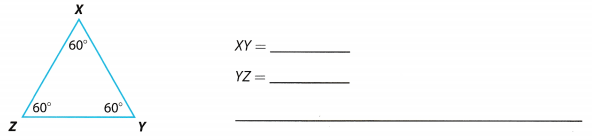Determine the side length of the triangle.
XY = 17 cm opposite side of ∠Z
YZ = 17 cm opposite side of ∠X

The triangle is an equilateral triangle with alt side lengths equal to 17 cm.

Area and Volume Equations

Essential Question
How can you use area and volume equations to solve real-world problems?

Exercises

Find the area of each figure. (Lessons 16.1, 16.2)

Question 1.b = 24
h = 12

Write equation of area of a parallelogram:
Area = b × h
Substitute values:
Area = 24 × 12
Evaluate:
Area = 288
Area of the parallelogram is 288 square inches

Question 2.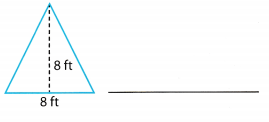Determine the area of the triangle.The area of the triangle is 32 ft2.

Find the missing measurement.

Question 3.b1 = 11, b2 = 14
Area = 62.5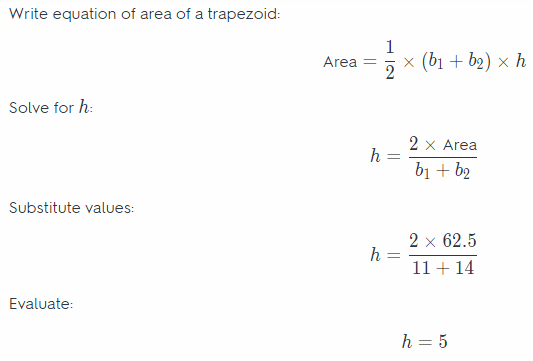Height of the given trapezoid is 5 meters.

Question 4.Solution to this example is given below
b = ? mm h = 4mm A = 26 mm2
A = $$\frac{1}{2}$$bh
26 = $$\frac{1}{2}$$(b)(4 mm) Substitute
26 = (2)b Multiply
$$\frac{26}{2}$$ = $$\frac{(2) b}{2}$$ Divide both sides by 2
13 = b Simplify
b = 13 mm Final solution

Find the volume of each rectangular prism.

Question 5.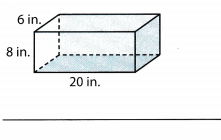l = 20
w = 6
h = 8
Write equation of volume of a rectangular prism:
Volume = l × w × h
Substitute values:
Volume = 20 × 6 × 8
Evaluate:
Volume = 960
Volume of the given rectangular prism is equal to 960 cubic inches.

Question 6.
A rectangular prism with a width of 7 units, a length of 8 units, and a height of 2 units ______________________
l = 8
w = 7
h = 2
Write equation of volume of a rectangular prism:
Volume = l × w × h
Substitute values:
Volume = 8 × 7 × 2
Evaluate:
Volume = 112
Volume of the given rectangular prism is equal to 112 cubic inches.

Question 7.
Jelani is ordering a piece of glass in the shape of a trapezoid to create a patio table top. Each square foot of glass costs $25. The trapezoid has base lengths of 5 feet and 3 feet and a height of 4 feet. Find the cost of the glass. (Lesson 16.1) Answer: Determine the area of the glass and the costThe glass costs$400

Question 1.
CAREERS IN MATH Theater Set Construction Ahmed and Karina are building scenery of the Egyptian pyramids out of plywood for a community play. The pyramids are represented by triangles on a rectangular base. The diagram shows the measurements of the piece of scenery.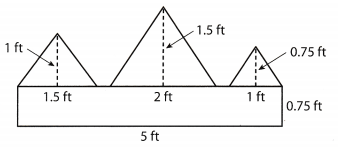a. They have one sheet of plywood, 3 ft by 6 ft. Will they be able to make the piece using this one sheet? Explain.
A = 3 6 area of the plywood
A= 18 ft2
Determine the area of the scenery.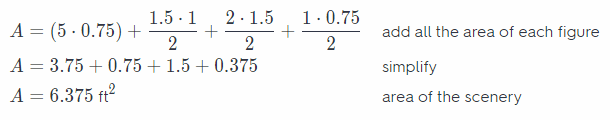Yes, they will be able to make the piece using only one sheet because the plywood is enough.

Determine the area of the completed piece.

b. How many square feet of plywood is in the completed piece? Show your work.
Determine the area of the completed piecec. The pyramids (the triangles) will be painted gray, and the base (the rectangle) will be painted black. How much of each paint color will they use, if one quart covers 45 square feet? Only one side of the model needs to be painted, but two coats of paint will be needed. Show your work. Round to the nearest hundredth of a square foot.
Determine the area of the rectangular base.
A = 5 0.75 area of the rectangular base
A = 3.75 . 2multiply by 2 for the paint needed
A = 7.5 ft2 area covered by the black paint
Determine the area of the triangles.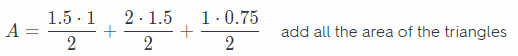A = 0.75 + 1.5 + 0.375 simplify
A = 2.625 . 2 multiply by 2 for the paint needed
A = 5.25 ft2 area covered by the gray paint

Determine the amount of paint to be used for the model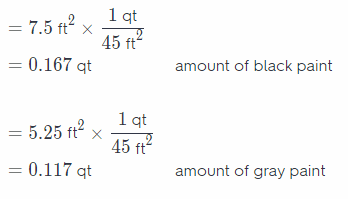Question 2.
Cassandra is making a design for a logo. One part of the design is a triangle with two congruent sides. She must draw the triangle with at least one side with length 6 centimeters, and at least one side with length 4 centimeters. Sketch two possible figures that Cassandra could use. Label the side lengths in both figures.
Figure that Cassandra could use.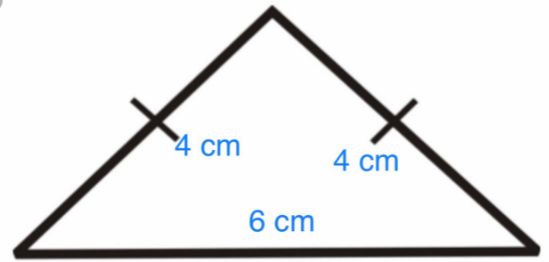Figure that Cassandra could use.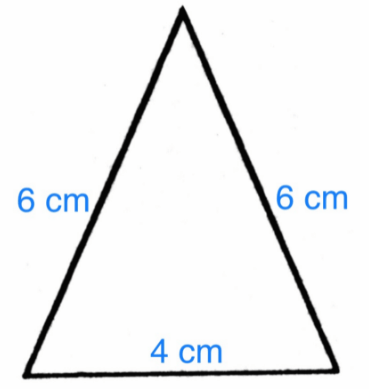The figure is an isosceles triangle with side lengths 6 cm – 6 cm – 4 cm or 4 cm – 4 cm – 6 cm.

Texas Go Math Grade 6 Unit 5 Mixed Review Texas Test Prep Answer Key

Selected Response

Question 1.
Part of a large wooden art project will be a triangle formed by joining three boards together. The artist has four boards that measure 16 feet, 11 feet, 7 feet, and 3 feet. Which board could not be used with two of the others to form a triangle?
(A) the 3-foot board
(B) the 7-foot board
(C) the 11-foot board
(D) the 16-foot board
(A) the 3-foot board

Explanation:
Find the sum of the two sides of the triangle then compare to the third side.
16 + 11 > 7 add the two sides and compare to the third side
27 > 7 the sum of the two sides is greater than the third side
16 + 7 > 11 add the two sides and compare to the third side
23 > 11 the sum of the two sides is greater than the third side
7 + 11 > 16 add the two sides and compare to the third side
17 > 16 the sum of the two sides is greater than the third side

The board that could not be used to form a triangle is A. the 3-foot board.

Question 2.
Which of these could be the value of x in the triangle below?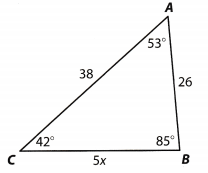(A) 5
(B) 6
(C) 8
(D) 10
(B) 6

Explanation:
Determine the opposite side length of the midsize angle.
a. 5x = 5 . 5 substitute for the given value of x
= 25
b. 5x = 5 . 6 substitute for the given value of x
= 30 opposite side length of the midsize angle
c. 5x = 5 8 substitute for the given value of x
= 40
d. 5x = 5 . 10 substitute for the given value of x
= 50
The value of x that will satisfy the given side lengths is B. 6.

Question 3.
What is the area of a trapezoid that has bases measuring 19 centimeters and 23 centimeters, and a height of 14 centimeters?
(A) 105 square centimeters
(B) 266 square centimeters
(C) 294 square centimeters
(D) 322 square centimeters
(C) 294 square centimeters

Explanation:
Solution to this example is given beLow
b1 = 19 b2 = 23 h = 14
Use the formula for area of a trapezoid
A = $$\frac{1}{2} h\left(b_{1}+b_{2}\right)$$
= $$\frac{1}{2} \cdot 14(19+23)$$ Substitute
= $$\frac{1}{2} \cdot 14(42)$$ Add inside the parentheses
= 7 . 42 Multiply $$\frac{1}{2}$$ and 14
= 294 square centimeters Multiply
C. This option is correct answer

Question 4.
What is the area of the triangle shown below?(A) 110.5 square inches
(B) 221 square inches
(C) 442 square inches
(D) 884 square inches
(B) 221 square inches

Explanation:
Find the area of the triangle.
b = 26 inches h = 17 inches
A = $$\frac{1}{2} b h$$
= $$\frac{1}{2}(26 \text { inches })(17 \text { inches })$$ Substitute
= 221 square inches Multiply
Area of the triangle is 221 square inches
B This option is correct answer

Question 5.
The trapezoid below has an area of 475 square meters.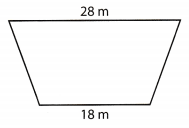Which equation could you solve to find the height of the trapezoid?
(A) 23h = 475
(B) 252h = 475
(C) 46h = 475
(D) 504h = 475
(A) 23h = 475

Explanation:
Data:
b1 = 18, b2 = 28
Area = 475

Write equation of area of a trapezoid:
Area = $$\frac{1}{2} \times\left(b_{1}+b_{2}\right) \times h$$
Substitute values:
475 = $$\frac{1}{2} \times(18+28) \times h$$
Simplify:
475 = 23h

Question 6.
A rectangular prism has a volume of 1,500 cubic centimeters. It has a length of 34 centimeters and a width of 22 centimeters. Which equation could be solved to find the height of the rectangular prism?
(A) 374h = 1,500
(B) 28h = 1,500
(C) 748h = 1,500
(D) 56h = 1,500
(C) 748h = 1,500

Explanation:
Data:
l = 34, w = 22
volume = 1500

Write equation of volume of a rectangular prism:
Volume = l × w × h
Substitute values:
1500 = 34 × 22 × h
Simplify:
1500 = 748h
Option C.

Question 7.
Which expression represents the sum of 59 and x?
(A) 59 + x
(B) 59 ÷ x
(C) 59x
(D) 59 – x
(A) 59 + x

Explanation:
Sum implies addition so the given statement is given by the expression: 59 + x.
Option A

Question 8.
Which number has more than two factors?
(A) 19
(B) 23
(C) 25
(D) 29
(C) 25

Explanation:
The number 25 has more than 2 factors. They are 1.5 and 25. The rest 3 numbers are prime in nature with only 1 and themselves as their factors.

Option C.

Question 9.
Which of the following statements about rational numbers is not correct?
(A) All whole numbers are also rational numbers.
(B) All integers are also rational numbers.
(C) All rational numbers can be written in the form $$\frac{a}{b}$$ where b ≠ 0.
(D) Rational numbers cannot be negative.
(D) Rational numbers cannot be negative.

Explanation:
Rational numbers are whole numbers, integers, and fractions in which positive and negative numbers included.
The incorrect statement is D. Rational numbers cannot be negative.

Gridded Response

Question 10.
What is the measure of the missing angle in a triangle that contains angle measures of 37° and 59°?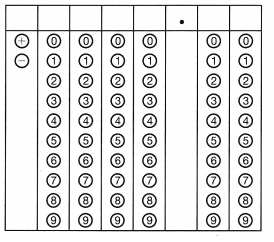Determine the unknown angle by adding the given angles and subtracting the result from 180°.
37° + 59° + x = 180° substitute for the sum of angle measures in triangle
96° + z = 180° sum of the two angles
96° + x – 96° = 180° – 96° subtract 96° from both sides of the equation
x = 84° measure of the unknown angle

The gridded response is 84.00°.

Hot Tip!
It is helpful to draw or redraw a figure. Answers to geometry problems may become clearer as you redraw the figure.

Question 11.
What is the measure, in degrees, of the missing angle in the triangle below?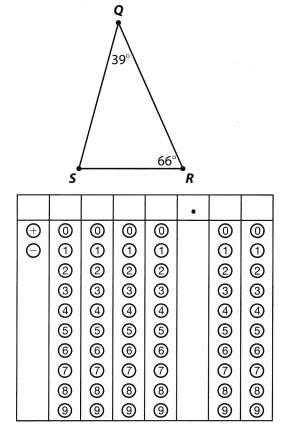Determine the unknown angle by adding the given angles and subtracting the result from 180°.
39 + 66° + x = 180° substitute for the sum of angle measures in triangle
105° + x = 180° sum of the two angles
105° + x – 105° = 180° – 105° subtract 105° from both sides of the equation
x = 75 measure of the unknown angle

The gridded response is 75.00

Question 12.
Janice wants to buy carpet for a trapezoid shaped room. The bases of the trapezoid are 12 feet and 14 feet, and the height is 15 feet. If the carpet she likes is $5.50 per square foot, how much will new carpet for the room cost in dollars?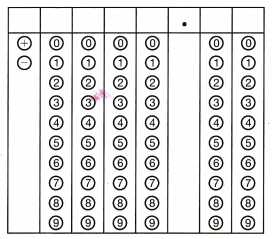Answer: Determine the area of the room and the cost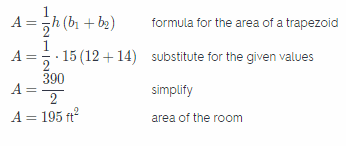Cost = 195 •$5.50 multiply the area by the cost per square foot
= $1,072.50 cost of the carpet for the room The gridded response is$1,072.50 which represents the cost of the carpet for the room.

Vocabulary Review

Use puzzle to preview key vocabulary from this unit. Unscramble the circled letters within found words to answer the riddle at the bottom of the page.• A triangle that has three congruent sides and three congruent angles. (Lesson 15-2)
• A triangle that has two congruent sides. (Lesson 15-3)
• A triangle that contains a right angle. (Lesson 15-2)
• A triangle that has no congruent sides. (Lesson 15-3)
• A quadrilateral where opposite sides are congruent and parallel. (Lesson 16-1)
• A quadrilateral in which all sides are congruent and opposite sides are parallel. (Lesson 16-1)

Q: Where does a mathematician go when she commits a crime?
A: __ __ __ __ ___!

Scroll to Top